Courses

Circular Motion MSQ

10 Questions MCQ Test Basic Physics for IIT JAM | Circular Motion MSQ

Description
Attempt Circular Motion MSQ | 10 questions in 45 minutes | Mock test for Physics preparation | Free important questions MCQ to study Basic Physics for IIT JAM for Physics Exam | Download free PDF with solutions
*Multiple options can be correct
QUESTION: 1

One end of a light string of length L is connected to a ball and the other end is connected to a fixed point O. The ball is released from rest at t = 0 with string horizontal and just taut. The ball then moves in vertical circular path as shown. The time taken by ball to go from position A to B is t1 and from B to lowest position C is t2. Let the velocity of ball at B is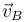and at C is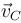respectively. If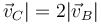then, which of the following is true.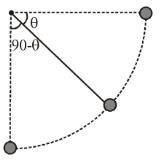Solution: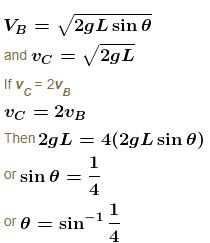Tangential acceleration is at = g cos θ which decrease with time.

Hence the plot of at versus time may be as shown in graph.

Area under graph in time interval t1 = vB – 0 = vB

Area under graph in the time interval t2 = vC – vB = vB

Hence the under graph in time t1 and t2 is same.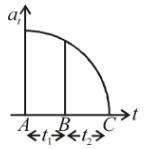∴  t1 < t2

∴ t1 < t2

The correct answers are: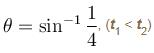*Multiple options can be correct
QUESTION: 2

A machine, in an amusement park, consists of a cage at the end of one arm, hinged at O. The cage revolves along a vertical circle of radius r (ABCDEFGH) about its hinge O, at constant linear speed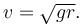The cage is so attached that the man of weight ‘w’ standing on a weighing machine, inside the cage, is always vertical. Then which of the following is/are correct.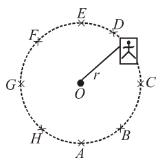Solution:

Let N be the normal reaction (Reading of the weighing machine)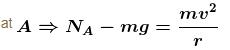Put v ∴  NA – mg = mg
⇒  NA = 2mg = 2W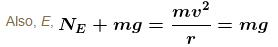hence  NA > NE by 2W
Now at GNG = mg = W = Nc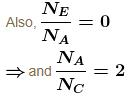The correct answers are: the weight reading at A is greater than the weight reading at E by 2 w, the weight reading at G = w, the ratio of the weight reading at E to that at A = 0, the ratio of the weight reading at A to that at C = 2

QUESTION: 3

A particle is moving in a circular path. The acceleration and momentum vectors at an instant of time are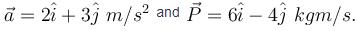Then which of the following statement is incorrect

Solution: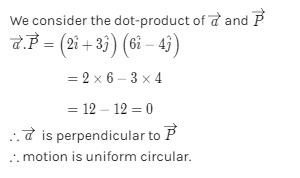*Multiple options can be correct
QUESTION: 4

A car driver going at some speed suddenly finds a wide wall at a distance r. To avoid hitting the wall he should.

Solution:

When he applies brakes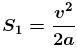if µ is the friction coefficient then a = µg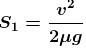when he takes turn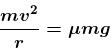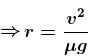Then we can see r < s1 hence driver can hit the wall when he take turn due to insufficient radius of curvature.

The correct answer is: apply the brakes

*Multiple options can be correct
QUESTION: 5

Two particles A and B separated by a distance 2R are moving counter clockwise along the same circular path of radius R each with uniform speed v. At time t = 0. A is given a tangential acceleration of magnitude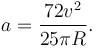Solution:

As when they collide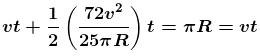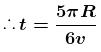Now angle covered by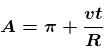Put t ∴ angle covered by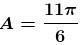The correct answers are: the time lapse for the two bodies to collide is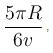the angle covered of A is 11π/6

*Multiple options can be correct
QUESTION: 6

A smooth rod PQ rotates in a horizontal plane about its mid point M which is h = 0.1 m vertically below a fixed point A at a constant angular velocity 14 rad/s. A light elastic string of natural length 0.1 m force constant 1.47 N/cm has one end fixed at A and its other end attached to a ring of mass m = 0.3 kg which is free to slide along the rod. When the ring is stationary relative to rod, then which of following is correct.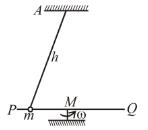Solution: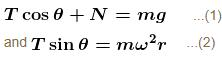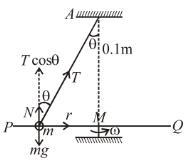but R = Kx
(K = 1.47 × 102 N/m)
Also  r = 0.1 tan θ
put Trm  and ω in equation (2)
We have cos θ = 3/5 and T = 9.8 N
The correct answers are: Tension in spring is 9.8 N, Inclination of string with vertical is cos–1(3/5)

*Multiple options can be correct
QUESTION: 7

On a circular table, A and B are moving on the circumference. Man A runs behind man B to catch him A runs with constant angular speed  ω1 with respect to table and B runs at constant tangential speed v2 with respect to ground. If it is found that the table rotates 30° in the opposite direction in every one second and the initial angular separation between A and B is 30°, then A catches B after : (Radius of table is 3 m).

Solution: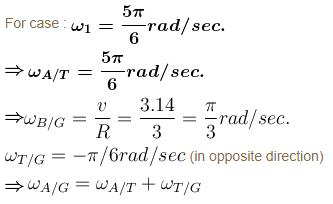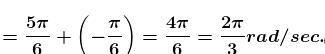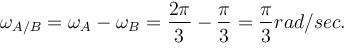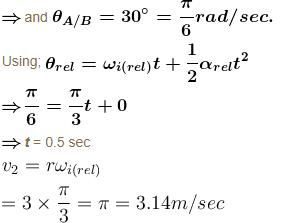The correct answers are: 0.5 sec, if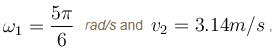A can not catch B within 0.5 sec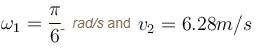*Multiple options can be correct
QUESTION: 8

A small sphere of mass m suspended by a thread is first taken aside so that the thread forms the right angle with the vertical and then released, then :

Solution: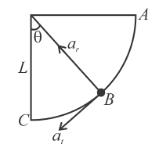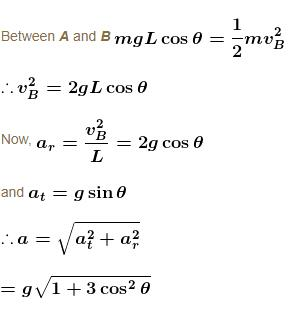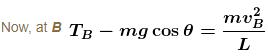By putting the value of VB

TB = 3mg cosθ

When total acceleration vector directed horizontally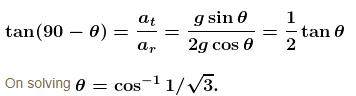The correct answers are: total acceleration of sphere as a function of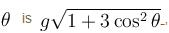thread tension as a function of θ is T = 3mg cos θ between the thread and the vertical at the moment when the total acceleration vector of the sphere is directed horizontal is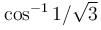*Multiple options can be correct
QUESTION: 9

A section of fixed smooth circular track of radius 20 m, in vertical plane is shown in the figure. A block is released from position A and leaves the track at B :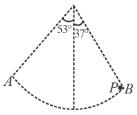Solution: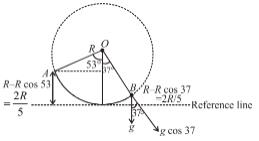By energy conservation between A and B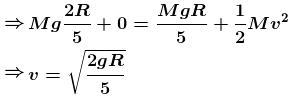Now, radius of curvature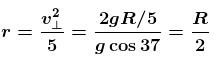The correct answers are: velocity at point B is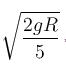Radius of curvature of trajectory when leaves point B is R/2

*Multiple options can be correct
QUESTION: 10

A particle is describing circular motion in a horizontal plane in contact with the smooth inside surface of a fixed right circular cone with its axis vertical and vertex down. The height of the plane of motion above the vertex is h and the semi-vertical angle of the cone is α. The period of revolution of the particle :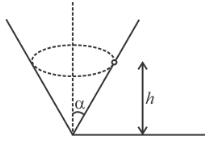Solution: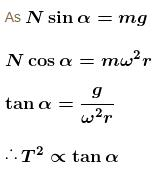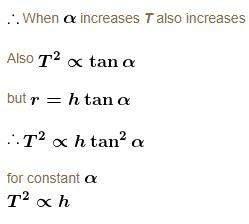Thus when h increases T also increases.
The correct answers are: increases as h increases, increases as α  increasesUse Code STAYHOME200 and get INR 200 additional OFF Use Coupon Code

Track your progress, build streaks, highlight & save important lessons and more!

Similar ContentRelated tests